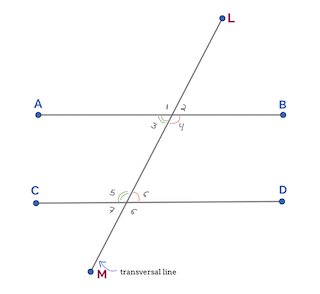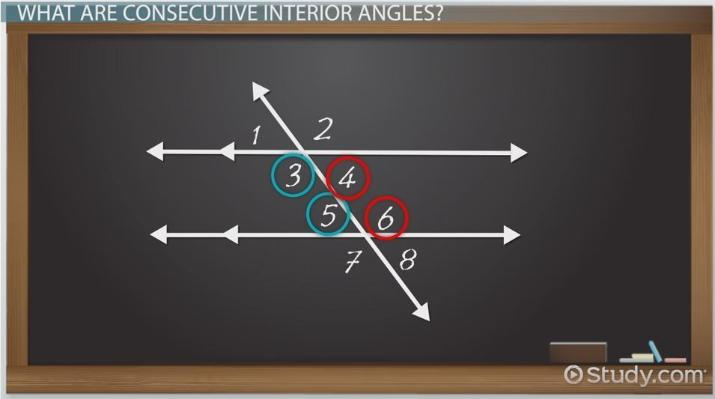# Consecutive Interior Angles

Courtney Hitchings, Yuanxin (Amy) Yang Alcocer
• Author
Courtney Hitchings

Courtney has 6+ years of high school math education experience. She received her BA in Math Secondary Education at the University of Delaware and her M.Ed in Curriculum and Instruction from Concorida University.

• Instructor
Yuanxin (Amy) Yang Alcocer

Amy has a master's degree in secondary education and has been teaching math for over 9 years. Amy has worked with students at all levels from those with special needs to those that are gifted.

Learn what are consecutive interior angles with definition and diagram. Understand the consecutive interior angles theorem with its applications through examples. Updated: 03/07/2022

Show

## What Are Consecutive Interior Angles?

Angles are created when two or more lines intersect, depending on how these lines cross, they will create different types of angles. In geometry, a pair of angles can relate to each other in a variety of ways. This helps identify them and use the theorem to solve problems related to angles. This lesson will focus on consecutive interior angles.

Consecutive interior angles are a pair of angles formed when a line, known as the transversal line, crosses two lines, parallel or non-parallel. The angles created on the inside of the two lines and are on the same side of the transversal line are known as the consecutive interior angles or co-interior angles for short.Looking at the image closely, one can identify the following:

The lines AB and CD are parallel lines

The line LM is a transversal line as it crossed lines AB and CD

{eq}\angle 3 {/eq} and {eq}\angle 5 {/eq} are co-interior angles

{eq}\angle 4 {/eq} and {eq}\angle 6 {/eq} are also co-interior angles

{eq}\angle 1 {/eq} and {eq}\angle 7 {/eq}\ are co-exterior angles

{eq}\angle 2 {/eq} and {eq}\angle 8 {/eq}\ are also co-exterior anglesAn error occurred trying to load this video.

Try refreshing the page, or contact customer support.

Coming up next: Exterior Angle Theorem: Definition & Formula

### You're on a roll. Keep up the good work!

Replay
Your next lesson will play in 10 seconds
• 0:00 What Are Consecutive…
• 0:44 Identifying…
• 1:40 The Theorem
• 2:23 Using the Theorem
• 3:21 Lesson Summary
Save Save

Want to watch this again later?

Timeline
Autoplay
Autoplay
Speed Speed

## Consecutive Interior Angles Theorem

The consecutive interior angle theorem states that if a transversal line intersects a pair of parallel lines, then the sum of the consecutive interior angles is equal to 180 degrees. Using the sample illustration to determine the location of the angles, one can see that:

{eq}\angle 3 {/eq} + {eq}\angle 5 {/eq} = 180 degrees

{eq}\angle 4 {/eq}+ {eq}\angle 6 {/eq} = 180 degrees

In geometry, it is essential to prove the theorems, to better understand them, hence the following is a simple way to prove the consecutive interior angle theorem:

Given that {eq}\overline{AB} {/eq} and {eq}\overline{CD} {/eq} are parallel lines and {eq}\overline{LM} {/eq} is a transversal line. We know that

{eq}\angle 2 + \angle 4 {/eq} = 180 degrees (because they form a linear pair)

{eq}\angle 2 = \angle 6 {/eq}(because of the corresponding angle theorem)

If we substitute {eq}\angle 2 {/eq}with {eq}\angle 6 {/eq} we will get

{eq}\angle 6 + \angle 4 {/eq} = 180 degrees and therefore proving the consecutive interior angles theorem.

### Converse of Consecutive Interior Angles Theorem

The converse of consecutive interior angles theorem states that if two lines are crossed by a transversal line and the consecutive interior angles are supplementary, which means when added they equal 180o, then the two lines, that were crossed, are parallel to one another.

The converse of consecutive interior angles theorem is used to identify if the lines that are crossed by a transversal line are parallel to each other or not.

### Are Consecutive Interior Angles Congruent?

Two angles are considered to be congruent if they have the same measurement or degree to one another. Consecutive interior angles are not congruent, but they are supplemental angles. Meaning the sum of the consecutive interior angles is equal to 180 degrees when a transversal line crosses through two parallel lines.

It is important to note that this might not be true if a transversal line crosses through two non-parallel lines.

### Supplementary Consecutive Interior Angles

Supplementary angles are two angles that when added together will equal 180o. It's important to note that not all consecutive interior angles are supplementary, meaning it doesn't always have to equal to 180 degrees. Consecutive interior angles are considered supplementary only when a transversal line crosses two parallel lines.

If a transversal line cross two non-parallel lines, then the consecutive interior angles formed will not be supplemental, meaning their sum will not equal to 180 degress.

To unlock this lesson you must be a Study.com Member.

#### Are consecutive interior angles congruent?

Consecutive Interior Angles are not congruent, they are supplemental and add up to 180 degrees if the two lines are crossed by the transversal line as parallel to one another.

#### What is the consecutive exterior angles theorem?

The consecutive interior angle theorem states that if a transversal line intersects a pair of parallel lines, then the sum of the consecutive interior angles is equal to 180o.

#### How do you know if angles are consecutive interior angles?

Two angles are consecutive interior angles if they follow these 2 properties. The two angles are both on the same side of a transversal AND they are both inside the two lines being crossed.

#### Do consecutive interior angles add up to 180?

Consecutive Interior Angles add up to 180 degrees, only if the two lines being crossed by a transversal line are parallel to one another.

### Register to view this lesson

Are you a student or a teacher?